﻿ 基于变增益滑动模态的无人潜水器高度控制
 舰船科学技术2019, Vol. 41Issue (8): 59-63PDF

Gain switching SMC-based altitude control of remotely operated vehicles
ZHUANG Peng, FENG Zheng-ping, BI An-yuan, HU Guo-dong
School of Naval Architecture, Ocean and Civil Engineering, Collaborative Innovation Center for Advanced Ship and Deep Sea Exploration, Shanghai Jiaotong University, Shanghai 200240, China
Abstract: Despite some successful applications of sliding mode control (SMC) in motion control of underwater vehicles, the chattering phenomenon of SMC is undesirable for control engineers and remain unresolved. A gain switching SMC which adjusts the output gain of the controller adaptively to suppress chattering is proposed in this paper. Moreover, to implement the state-feedback based scheme in altitude control of a remotely operated vehicle (ROV), a nonlinear state observer is adopted, which estimates the precise altitude and vertical velocity of ROV with higher update rate and reliability. The proposed approach is verified by tank tests.
Key words: sliding mode control     state observer     chattering     remotely operated vehicles
0 引　言

1 潜水器动力学建模

 \left\{ {\begin{aligned} & {\dot z = w}, \\ &{\dot w = \frac{1}{{m - {Z_{\dot w}}}}\left( {{Z_w} + {Z_{w\left| w \right|}}\left| w \right|} \right)w + \frac{1}{{m - {Z_{\dot w}}}}{T_z}} , \end{aligned}} \right. (1)

 \left\{ {\begin{aligned} & {\dot h = - w}, \\ & {\dot w = \frac{1}{{m - {Z_{\dot w}}}}\left( {{Z_w} + {Z_{w\left| w \right|}}\left| w \right|} \right)w + \frac{1}{{m - {Z_{\dot w}}}}{T_z}} {\text{。}} \end{aligned}} \right. (2)
2 控制器设计及稳定性分析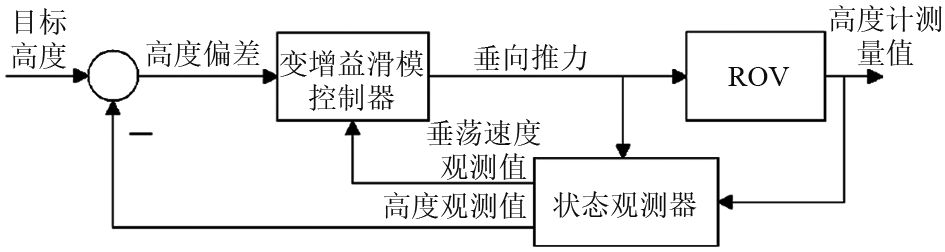图 1 控制系统结构图 Fig. 1 Structure of control system

2.1 变增益滑模控制器设计

 $s = \dot e + \lambda e,$ (3)

 $\dot s = - ksat\left( s \right), k > 0,$ (4)

 ${T_z} = - \left( {{Z_w} + {Z_{w\left| w \right|}}\left| w \right|} \right)w + \left( {m - {Z_{\dot w}}} \right)\left[ { - \lambda w + ksat\left( s \right)} \right]{\text{。}}$ (5)

 $T_z' = \sigma {T_z},$ (6)

 \sigma = \left\{ {\begin{aligned} & {{\sigma _1}, {\rm{when}}\left| s \right| > \Delta }, \\ & {{\sigma _2}, {\rm{when}}\left| s \right| \leqslant \Delta {\rm{and}}\left| e \right| \leqslant 0.1m} {\text{。}} \end{aligned}} \right. (7)
2.2 变增益滑模控制器稳定性分析

 ${T_z}^{'} = - \left( {{Z_w} + {Z_{w\left| w \right|}}\left| w \right|} \right)w + \left( {m - {Z_{\dot w}}} \right)\left[ { - \lambda 'w + k'sat\left( s \right)} \right],$ (8)

2.3 状态观测器设计

 \left\{ {\begin{aligned} & {\dot x = f\left( x \right) + g\left( x \right)U\left( t \right)}, \\ & {y = h\left( x \right)} , \end{aligned}} \right. (9)

 $f\left( x \right) = {\left[ { - {x_2}, \frac{1}{{m - {Z_{\dot w}}}}\left( {{Z_w} + {Z_{w\left| w \right|}}\left| {{x_2}} \right|} \right){x_2}} \right]^{\rm T}},$ (10)
 $g\left( x \right) = {\left[ {0, \frac{1}{{m - {Z_{\dot w}}}}} \right]^{\rm T}}, h\left( x \right) = {x_1}{\text{。}}$ (11)

 \left\{ {\begin{aligned} & {\dot {\hat x} \left( t \right) \!=\! f\left( {\hat x\left( t \right)} \right) \!+\! g\left( {\hat x\left( t \right)} \right)U\left( t \right) + {{\left[ {Q\left( {\hat x\left( t \right)} \right)} \right]}^{ - 1}}K\left[ {y\left( t \right) \!-\! h\left( {\hat x\left( t \right)} \right)} \right]}, \\ & {\hat x\left( 0 \right) = \bar x}, \end{aligned}} \right. (12)

 $Q\left( x \right) = \frac{{{\rm{d}}\varPhi \left( x \right)}}{{{\rm{d}}x}},$ (13)

 $\varPhi \left( x \right) \!\approx\! \zeta \!=\! \left[\! {\begin{array}{*{20}{c}} {{\zeta _1}} \\ {{\zeta _2}} \end{array}} \!\right] \!=\! \left[\! {\begin{array}{*{20}{c}} {h\left( x \right)} \\ {{L_f}h\left( x \right)} \end{array}} \!\right] \!=\! \left[\! {\begin{array}{*{20}{c}} {{x_1}} \\ { - {x_2}} \end{array}} \!\right], K \!=\! \left[\! {\begin{array}{*{20}{c}} {{K_1}} \\ {{K_2}} \end{array}} \!\right],$ (14)

 $Q\left( x \right) = \frac{{{\rm{d}}\varPhi \left( x \right)}}{{{\rm{d}}x}} = \left[ {\begin{array}{*{20}{c}} 1 & 0 \\ 0 & { - 1} \end{array}} \right]{\text{。}}$ (15)

 $\left( {\mu - {\mu _1}} \right)\left( {\mu - {\mu _2}} \right) = \mu + {K_1}\mu + {K_2},$ (16)

μ1μ2根据Re{μi}<–γi = 1，2选取后代入式（16）可计算得到Kγ需满足

 $\gamma \geqslant \left| {\frac{{{\rm{d}}a\left( {{\zeta _2}} \right)}}{{{\rm{d}}{\zeta _2}}}} \right|,$ (17)

3 实验验证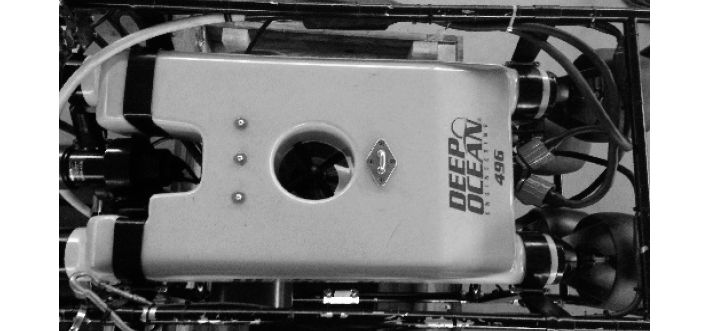图 2 无人遥控潜水器DOE ROV Fig. 2 DOE HD2+2 Remote Operated Vehicle

DOE HD2+2 ROV的主要尺寸数据如表1所示。该潜水器在水下具有零浮力，配有一个最大推力为7 kg的垂向推进器。此外还配有高度传感器、惯性导航传感器等设备，用于测量ROV高度和姿态等数据。表 1 无人遥控潜水器DOE ROV的主要尺寸参数 Tab.1 Parameters of DOE ROV表 2 无人潜水器DOE ROV的主要水动力参数 Tab.2 Hydrodynamic coefficients of DOE ROV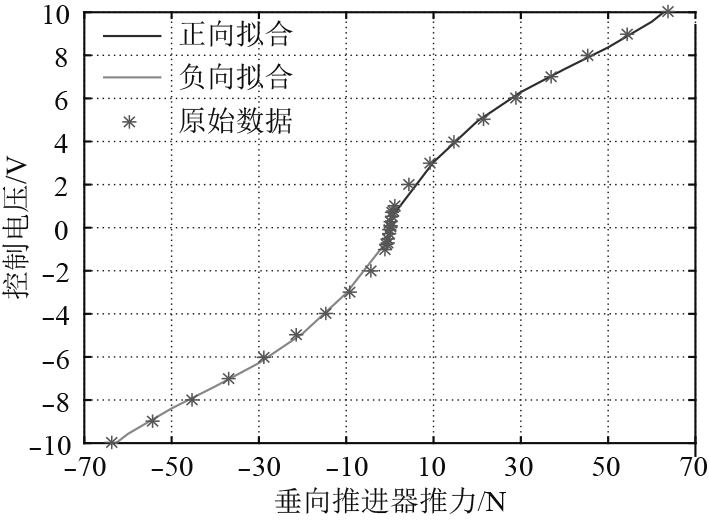图 3 控制电压与推进器推力的关系 Fig. 3 Forward and reverse propeller force applied voltage relations

3.1 状态观测器性能实验验证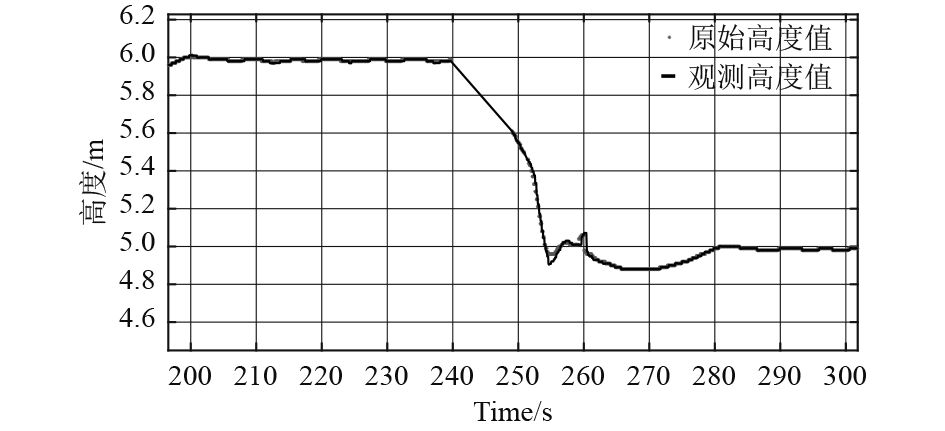图 4 状态观测器对ROV高度的观测效果 Fig. 4 Experimental state observer results for altitude of ROV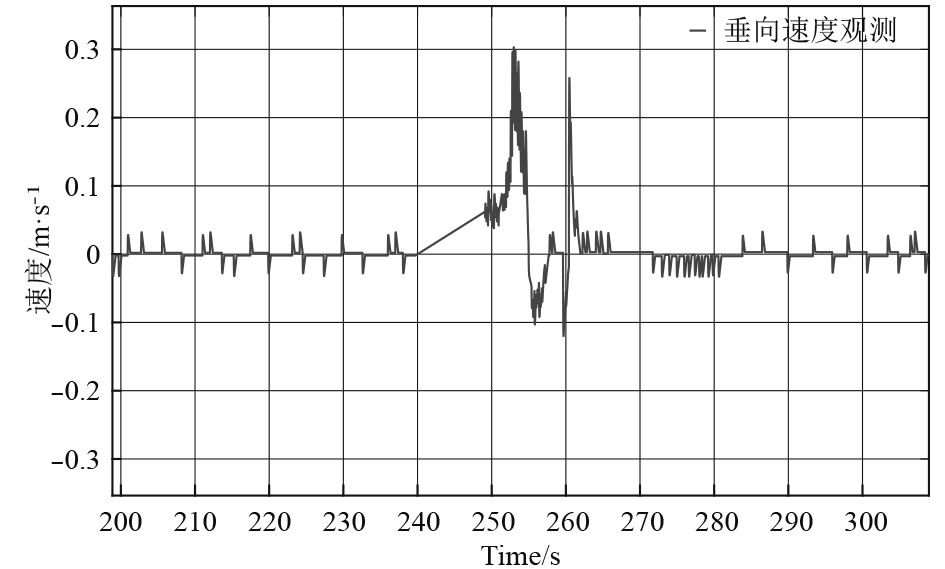图 5 状态观测器对ROV垂向速度的观测效果 Fig. 5 Experimental state observer results for vertical speed of ROV
3.2 变增益SMC高度控制性能实验验证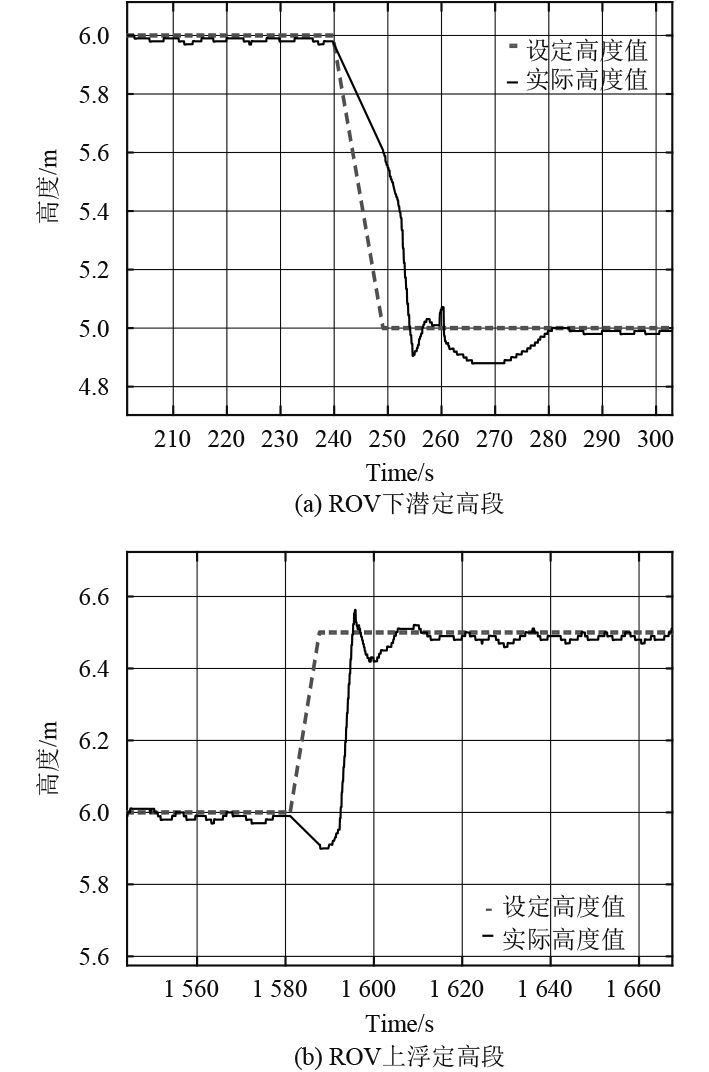图 6 ROV高度控制效果 Fig. 6 Experimental altitude control results of ROV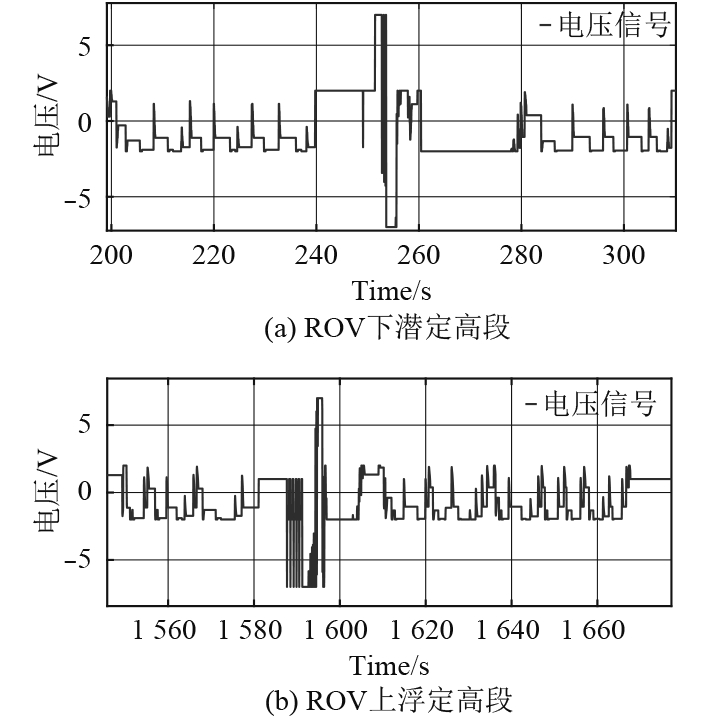图 7 垂向推进器控制信号输入 Fig. 7 Signal input of vertical thruster
4 结　语

  CHU Z, ZHU D, YANG S X. Observer-based adaptive neural network trajectory tracking control for remotely operated vehicle[J]. IEEE Transactions on Neural Networks & Learning Systems, 2017, 28(7): 1633-45.  TREBI-OLLENNU A, STACEY B A, WHITE B A. A multivariable decoupling design of an ROV depth control system (A Direct Adaptive Fuzzy SMC Approach)[J]. Journal of dynamic systems, measurement, and control, 1997, 119(1): 89-94. DOI:10.1115/1.2801222  MUÑOZ-VÁZQUEZ A J, RAMÍREZ-RODRÍGUEZ H, PARRA-VEGA V, et al. Fractional sliding mode control of underwater ROVs subject to non-differentiable disturbances[J]. International Journal of Control Automation & Systems, 2016, 15(1): 1-8.  LIANG X, QU X, HOU Y, et al. Three-dimensional path following control of underactuated autonomous underwater vehicle based on damping backstepping[J]. International Journal of Advanced Robotic Systems, 2017, 14(4): 1-9.  WANG Y H, WU Q X, LIU X. Adaptive fuzzy sliding mode control for MIMO nonaffine dutch-roll system[J]. Journal of Dynamic Systems Measurement & Control, 2017, 139(10): 101-9.  SONG B K, AN J H, CHOI S B. A new fuzzy sliding mode controller with a disturbance estimator for robust vibration control of a semi-active vehicle suspension system[J]. Applied Sciences, 2017, 10(7): 1053-73.  TONG S, LI H X. Fuzzy adaptive sliding-mode control for MIMO nonlinear systems[J]. IEEE Transactions on Fuzzy Systems, 2003, 11(3): 354-60. DOI:10.1109/TFUZZ.2003.812694  Y X, N F-C. Genetic algorithm based sliding mode control in the leader/follower satellites pair maintenance[J]. Advances in the Astronautical Sciences, 2004, 116: 1-16.  EDWARDS C, SPURGEON S K. Sliding mode control: theory and applications[M]. Crc Press, 1998.  YOUNG K D, UTKIN V I, OZGUNER U. A control engineer's guide to sliding mode control[J]. IEEE Transactions on Control Systems Technology, 1999, 7(3): 328-42. DOI:10.1109/87.761053  SOYLU S, BUCKHAM B J, PODHORODESKI R P. A chattering-free sliding-mode controller for underwater vehicles with fault-tolerant infinity-norm thrust allocation[J]. Ocean Engineering, 2008, 35(16): 1647-59. DOI:10.1016/j.oceaneng.2008.07.013  CICCARELLA G, MORA M D, GERMANI A. A Luenberger-like observer for nonlinear systems[J]. International Journal of Control, 1993, 57(3): 537-56. DOI:10.1080/00207179308934406  许孟孟, 冯正平, 毕安元. 复杂外形潜水器的动力学建模[J]. 舰船科学技术, 2017, 39(9): 23-8. DOI:10.3404/j.issn.1672-7649.2017.09.005  许孟孟. 具有复杂外形的ROV动力学建模及控制[D]. 上海: 上海交通大学, 2017.